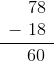# ISEE Lower Level Quantitative : How to subtract

## Example Questions

### Example Question #371 : Isee Lower Level (Grades 5 6) Quantitative Reasoning

The bowl iscentimeters long and the plate is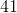centimeters long. How much longer is the plate than the bowl?centimeterscentimeterscentimeterscentimeterscentimeterscentimeters

Explanation:

This is a subtraction problem because we want to know how much longer the plate is compared to the bowl, or the difference in their length.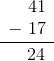### Example Question #372 : Isee Lower Level (Grades 5 6) Quantitative Reasoning

The swing set iscentimeters tall and the tree iscentimeters tall. How much taller is the tree than the swing set?centimeterscentimeterscentimeterscentimeterscentimeterscentimeters

Explanation:

This is a subtraction problem because we want to know how much taller the tree is compared to the swing set, or the difference in their height.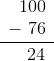### Example Question #373 : Isee Lower Level (Grades 5 6) Quantitative Reasoning

The remote iscentimeters long and the TV iscentimeters long. How much longer is the TV than the remote?centimeters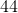centimeters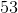centimeterscentimeterscentimeterscentimeters

Explanation:

This is a subtraction problem because we want to know how much longer the TV is compared to the remote, or the difference in their length.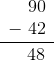### Example Question #374 : Isee Lower Level (Grades 5 6) Quantitative Reasoning

The dog’s body iscentimeters long and his tail is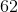centimeters long. How much longer is his body than his tail?centimeterscentimeterscentimeterscentimeterscentimeterscentimeters

Explanation:

This is a subtraction problem because we want to know how much longer the dog's body is compared to his tail, or the difference in their length.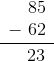### Example Question #381 : Isee Lower Level (Grades 5 6) Quantitative Reasoning

The coffee cup iscentimeters tall and the water glass iscentimeters tall. How much taller is the water glass than the coffee cup?centimeterscentimeterscentimeterscentimeterscentimeterscentimeters

Explanation:

This is a subtraction problem because we want to know how much taller the water glass is compared to the coffee cup, or the difference in their height.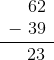### Example Question #382 : Isee Lower Level (Grades 5 6) Quantitative Reasoning

The cat iscentimeters long and the dog iscentimeters long. How much longer is the dog than the cat?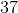centimeterscentimeterscentimeterscentimeterscentimeterscentimeters

Explanation:

This is a subtraction problem because we want to know how much longer the dog is compared to the cat, or the difference in their length.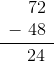### Example Question #383 : Isee Lower Level (Grades 5 6) Quantitative Reasoning

The cat iscentimeters long and the dog is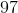centimeters long. How much longer is the dog than the cat?centimeterscentimeters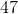centimeterscentimeterscentimeterscentimeters

Explanation:

This is a subtraction problem because we want to know how much longer the dog is compared to the cat, or the difference in their length.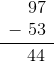### Example Question #384 : Isee Lower Level (Grades 5 6) Quantitative Reasoning

The bowl iscentimeters long and the plate iscentimeters long. How much longer is the plate than the bowl?centimeterscentimeterscentimeterscentimeterscentimeterscentimeters

Explanation:

This is a subtraction problem because we want to know how much longer the plate is compared to the bowl, or the difference in their length.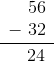### Example Question #385 : Isee Lower Level (Grades 5 6) Quantitative Reasoning

The swing set is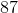centimeters tall and the tree is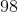centimeters tall. How much taller is the tree than the swing set?centimeterscentimeterscentimeterscentimeterscentimeterscentimeters

Explanation:

This is a subtraction problem because we want to know how much taller the tree is compared to the swing set, or the difference in their height.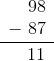### Example Question #386 : Isee Lower Level (Grades 5 6) Quantitative Reasoning

The remote iscentimeters long and the TV is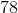centimeters long. How much longer is the TV than the remote?centimeters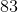centimeterscentimeters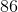centimeters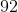centimeterscentimeters